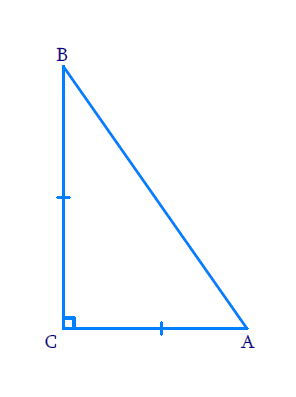# Ex.6.5 Q5 Triangles Solution - NCERT Maths Class 10

Go back to  'Ex.6.5'

## Question

$$ABC$$ is an isosceles triangle with $${AC = BC}$$. If $$A B^{2}=2 A C^{2}$$ prove that $$ABC$$ is a right triangle.

Diagram## Text Solution

Reasoning:

As we know ,In a triangle, if square of one side is equal to the sum of the squares of the other two sides, then the angle opposite the first side is a right angle.

Steps:

In $$\Delta A B C$$

$AC = BC$

And

\begin{align}A B^{2} &=2 A C^{2} \\ &=A C^{2}+A C^{2} \\ A B^{2} &=A C^{2}+B C^{2} \quad[\because A C=B C] \end{align}

$$\Rightarrow \angle A C B=90^\circ$$

\begin{align}\Rightarrow \Delta A B C \end{align} is a right triangle

Learn from the best math teachers and top your exams

• Live one on one classroom and doubt clearing
• Practice worksheets in and after class for conceptual clarity
• Personalized curriculum to keep up with school